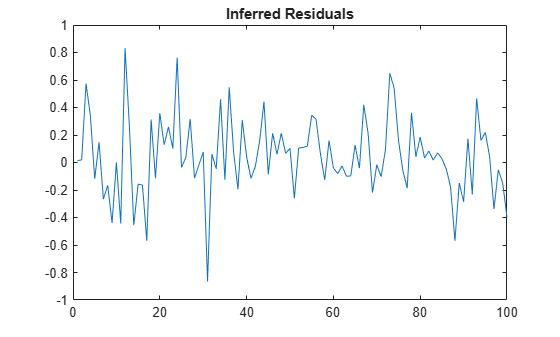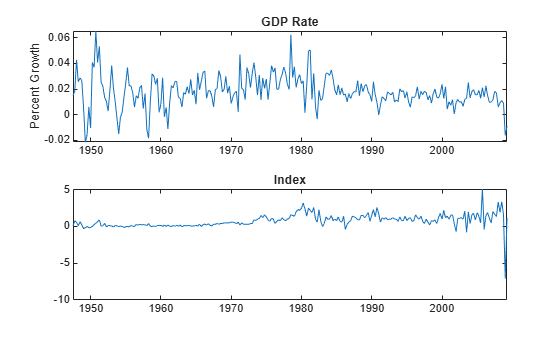# infer

Class: regARIMA

Infer innovations of regression models with ARIMA errors

## Syntax

E = infer(Mdl,Y) [E,U,V,logL] = infer(Mdl,Y) [E,U,V,logL] = infer(Mdl,Y,Name,Value) 

## Description

E = infer(Mdl,Y) infers residuals of a univariate regression model with ARIMA time series errors fit to response data Y.

[E,U,V,logL] = infer(Mdl,Y) additionally returns the unconditional disturbances U, the innovation variances V, and the loglikelihood objective function values logL.

[E,U,V,logL] = infer(Mdl,Y,Name,Value) returns the output arguments using additional options specified by one or more Name,Value pair arguments.

## Input Arguments

expand all

Regression model with ARIMA errors, specified as a regARIMA model returned by regARIMA or estimate.

The properties of Mdl cannot contain NaNs.

Response data, specified as a numeric column vector or numeric matrix. If Y is a matrix, then it has numObs observations and numPaths rows.

infer infers the residuals (estimated innovations) and unconditional disturbances of Y. Y represents the time series characterized by Mdl, and it is the continuation of the presample series Y0.

• If Y is a column vector, then it represents one path of the underlying series.

• If Y is a matrix, then it represents numObs observations of numPaths paths of an underlying time series.

infer assumes that observations across any row occur simultaneously. The last observation of any series is the latest.

Data Types: double

### Name-Value Arguments

Specify optional pairs of arguments as Name1=Value1,...,NameN=ValueN, where Name is the argument name and Value is the corresponding value. Name-value arguments must appear after other arguments, but the order of the pairs does not matter.

Before R2021a, use commas to separate each name and value, and enclose Name in quotes.

Presample innovations that have mean 0 and provide initial values for the ARIMA error model, specified as the comma-separated pair consisting of 'E0' and a numeric column vector or numeric matrix.

• If E0 is a column vector, then it is applied to each inferred path.

• If E0 is a matrix, then it requires at least numPaths columns. If E0 contains more columns than required, then infer uses the first numPaths columns.

• E0 must contain at least Mdl.Q rows. If E0 contains extra rows, then infer uses the latest presample innovations. The last row contains the latest presample innovation.

By default, infer sets the necessary observations to 0.

Data Types: double

Presample unconditional disturbances that provide initial values for the ARIMA error model, specified as the comma-separated pair consisting of 'U0' and a numeric column vector or numeric matrix.

• If U0 is a column vector, then it is applied to each inferred path.

• If U0 is a matrix, then it requires at least numPaths columns. If U0 contains more columns than required, then infer uses the first numPaths columns.

• U0 must contain at least Mdl.P rows. If U0 contains extra rows, then infer uses the latest presample unconditional disturbances. The last row contains the latest presample unconditional disturbance.

By default, infer backcasts for the necessary presample unconditional disturbances.

Data Types: double

Predictor data in the regression model, specified as the comma-separated pair consisting of 'X' and a numeric matrix.

The columns of X are separate, synchronized time series, with the last row containing the latest observations. The number of rows of X should be at least the length of Y. If the number of rows of X exceeds the number required, then infer uses the latest observations.

By default, infer does not include a regression component in the model regardless of the presence of regression coefficients in Mdl.

Data Types: double

Notes

• NaNs in Y, X, E0, and U0 indicate missing values and infer removes them. The software merges the presample data sets (E0 and U0), then uses list-wise deletion to remove any NaNs. infer similarly removes NaNs from the effective sample data (X and Y). Removing NaNs in the data reduces the sample size, and can also create irregular time series.

• infer assumes that you synchronize presample data such that the latest observation of each presample series occurs simultaneously.

• All predictors (that is, columns in X) are associated with each response path in Y.

• V is equal to the variance in Mdl.

## Output Arguments

expand all

Inferred residuals (estimated innovations of the unconditional disturbances), returned as a numeric matrix. E has numObs rows and numPaths columns.

Inferred residuals are

${e}_{t}={\stackrel{^}{u}}_{t}-{\varphi }_{1}{\stackrel{^}{u}}_{t-1}-...-{\varphi }_{P}{\stackrel{^}{u}}_{t-P}-{\theta }_{1}{e}_{t-1}-...-{\theta }_{Q}{e}_{t-Q}$

${\stackrel{^}{u}}_{t}$ is row t of the inferred unconditional disturbances U, ϕj is composite autoregressive coefficient j, and θk is composite moving average coefficient k.

Data Types: double

Inferred unconditional disturbances, returned as a numeric matrix. U has numObs rows and numPaths columns.

Inferred unconditional disturbances are

${\stackrel{^}{u}}_{t}={y}_{t}-c-{x}_{t}\beta .$

yt is row t of the response data Y, xt is row t of the predictor data X, c is the model intercept Mdl.Intercept, and β is the vector of regression coefficients Mdl.Beta.

Data Types: double

Inferred variances, returned as a numeric matrix. V has numObs rows and numPaths columns.

All elements in V are equal to Mdl.Variance.

Data Types: double

Loglikelihood objective function values associated with the model Mdl, returned as a numeric vector. logL has numPaths elements associated with the corresponding path in Y.

Data Types: double

## Examples

expand all

Forecast responses from the following regression model with ARMA(2,1) errors over a 30-period horizon:

$\begin{array}{l}\begin{array}{c}{y}_{t}={X}_{t}\left[\begin{array}{c}0.1\\ -0.2\end{array}\right]+{u}_{t}\\ {u}_{t}=0.5{u}_{t-1}-0.8{u}_{t-2}+{\epsilon }_{t}-0.5{\epsilon }_{t-1},\end{array}\end{array}$

where ${\epsilon }_{t}$ is Gaussian with variance 0.1.

Specify the regression model with ARIMA errors. Simulate responses from the model and two predictor series.

Mdl = regARIMA('Intercept', 0, 'AR', {0.5 -0.8}, ... 'MA',-0.5,'Beta',[0.1 -0.2], 'Variance',0.1); rng(1); % For reproducibility X = randn(100,2); y = simulate(Mdl,100,'X',X);

Infer, and then plot residuals. By default, infer backcasts for the necessary presample unconditional disturbances.

e = infer(Mdl,y,'X',X); figure plot(e) title('Inferred Residuals')Regress the log GDP onto the CPI using a regression model with ARMA(1,1) errors, and then examine the residuals.

Load the U.S. Macroeconomic data set and preprocess the data.

load Data_USEconModel; logGDP = log(DataTimeTable.GDP); dlogGDP = diff(logGDP); % For stationarity dCPI = diff(DataTimeTable.CPIAUCSL); % For stationarity T = length(dlogGDP); % Effective sample size

Fit a regression model with ARMA(1,1) errors.

Mdl = regARIMA(1,0,1); EstMdl = estimate(Mdl,dlogGDP,'X',dCPI);
 Regression with ARMA(1,1) Error Model (Gaussian Distribution): Value StandardError TStatistic PValue __________ _____________ __________ __________ Intercept 0.014776 0.0014627 10.102 5.4239e-24 AR{1} 0.60527 0.08929 6.7787 1.2124e-11 MA{1} -0.16165 0.10956 -1.4755 0.14009 Beta(1) 0.002044 0.00070616 2.8946 0.0037969 Variance 9.3578e-05 6.0314e-06 15.515 2.7338e-54 

Infer the residuals over all observations. By default, infer backcasts for the necessary unconditional disturbances.

e = infer(EstMdl,dlogGDP,'X',dCPI);

Plot the inferred residuals.

figure plot(1:T,e,[1 T],[0 0],'r') title('{\bf Inferred Residuals}')The residuals are centered around 0, but show signs of heteroscedasticity.

## References

 Box, G. E. P., G. M. Jenkins, and G. C. Reinsel. Time Series Analysis: Forecasting and Control. 3rd ed. Englewood Cliffs, NJ: Prentice Hall, 1994.

 Davidson, R., and J. G. MacKinnon. Econometric Theory and Methods. Oxford, UK: Oxford University Press, 2004.

 Enders, W. Applied Econometric Time Series. Hoboken, NJ: John Wiley & Sons, Inc., 1995.

 Hamilton, J. D. Time Series Analysis. Princeton, NJ: Princeton University Press, 1994.

 Pankratz, A. Forecasting with Dynamic Regression Models. John Wiley & Sons, Inc., 1991.

 Tsay, R. S. Analysis of Financial Time Series. 2nd ed. Hoboken, NJ: John Wiley & Sons, Inc., 2005.